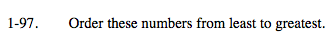### Home > MC2 > Chapter 1 > Lesson 1.2.4 > Problem1-97

1-97.Note: There is more than one approach to solve this problem.

Remember, negative numbers are less than positive numbers.

$1.1,\; 0,\; \frac{5}{8}, \;2, \;0.4, \;\frac{5}{3}, \;-2, \;\frac{1}{2}$

Change the above numbers into either all fractions or all decimals.

$\text{Using a calculator, } \frac{5}{8} \text{ is equal to 0.625.}$

Now change the rest of the fractions to decimals.

Use the eTool below to find the decimal equivalent for the numbers.
Click the link at right for the full version of the eTool: MC2 1-97 HW eTool